• BOOK MARK
• HOME
• CART
• TODAY VIEW
코웨이
Total 463 items in this category
검색결과 정렬• CHPI-610L 역삼투압(R/O)포함 8개 세트
[4+2+R/O1+1]
1년 세트
• 66,000원
63,000원• CHPI-610L 역삼투압(R/O)포함 4개 세트
[1+1+UF1+1]
• 51,000원
48,000원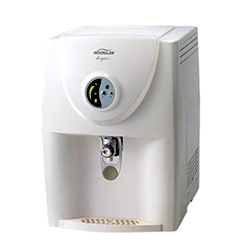• 코웨이(웅진)
CP-01CL
• 49,000원
45,000원
• 정수기는 판매하지 않습니다.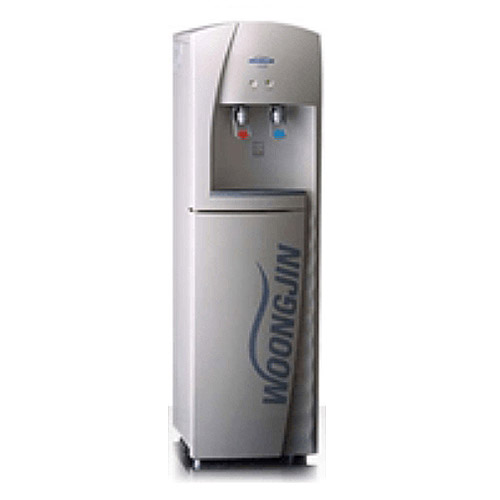• 코웨이(웅진)
CHP-8800
• 호환 필터
• 정수기는 판매하지 않습니다.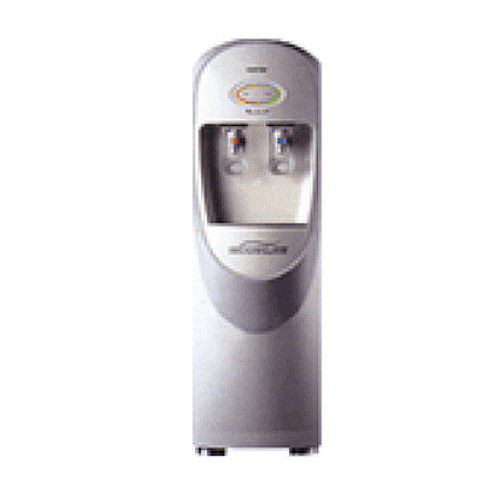• 코웨이(웅진)
WJCHP-8500
• 호환 필터
• 정수기는 판매하지 않습니다.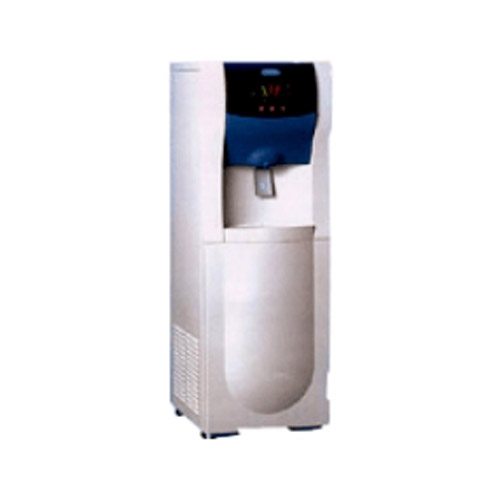• 코웨이(웅진)
WJCHP-8100
• 호환 필터
• 정수기는 판매하지 않습니다.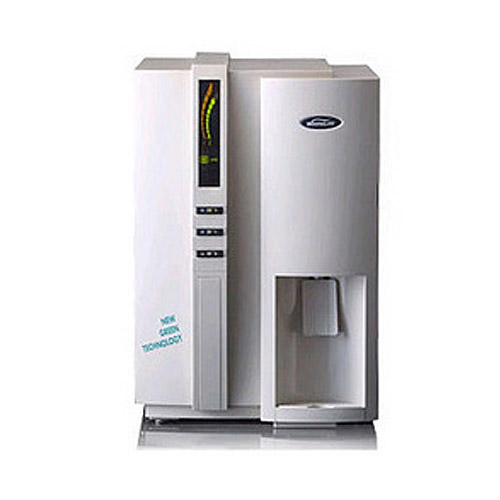• 코웨이(웅진)
PHP-7100
• 호환 필터
• 정수기는 판매하지 않습니다.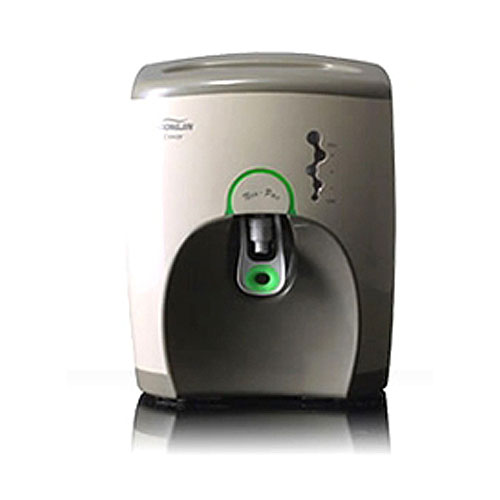• 코웨이(웅진)
P-5200(Neo-Pac)
• 호환 필터
• 정수기는 판매하지 않습니다.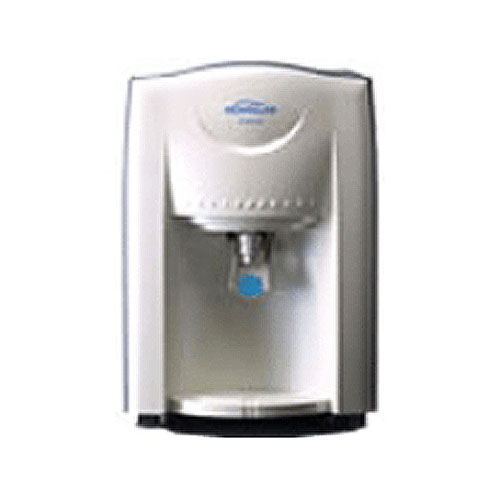• 코웨이(웅진) P-5100
• 호환 필터
• 정수기는 판매하지 않습니다.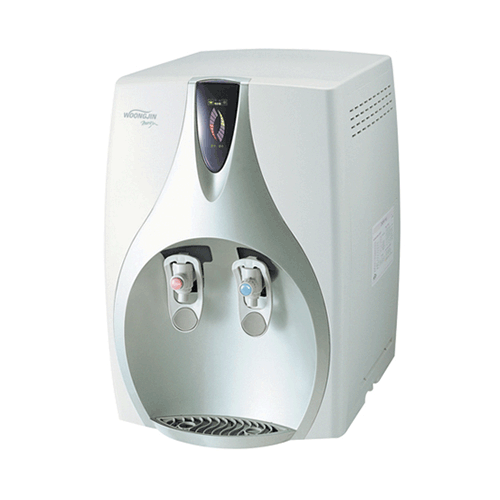• 코웨이(웅진)
CHP-01BL(누리수)
• 호환 필터
• 정수기는 판매하지 않습니다.• 코웨이(웅진) CHP-01BR(누리수)
• 호환 필터
• 정수기는 판매하지 않습니다.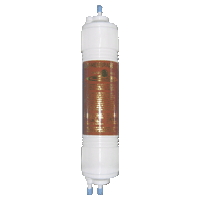• P-07QL호환
역삼투압(R/O)필터
• 53,000원• P-07QL호환
후처리(포스트)카본필터
• 10,000원• P-07QL호환
침전+프리(네오복합)필터
• 13,000원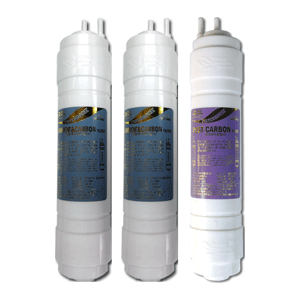• P-07QL호환
멤브레인제외
3개세트[2+1]
• 36,000원
33,000원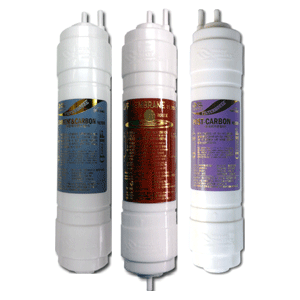• P-07QL호환
역삼투압(R/O)포함
3개세트[1+RO1+1]
• 76,000원
74,000원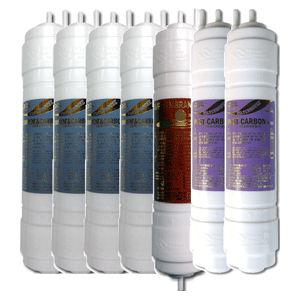• P-07QL 호환
역삼투압(R/O)포함
7개세트[4+RO1+2]
• 125,000원
115,000원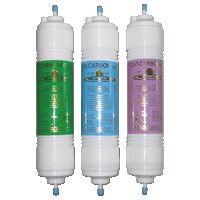• WJCHP-8050호환
멤브레인제외
3개셋트[1+1+0+1]
• 27,000원
25,000원• WJCHP-8050호환
멤브레인제외
3개셋트[1+1+0+1]
• 21,000원
19,000원• CHP-9100호환
멤브레인제외
3개세트[1+1+0+1]
• 27,000원
25,000원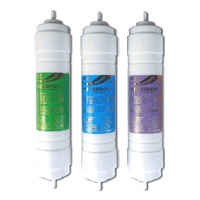• CHP-9100호환
멤브레인제외
3개세트[1+1+0+1]
• 21,000원
19,000원• WJCHP-9000호환
멤브레인제외
3개셋트[1+1+0+1]
• 26,000원
25,000원• CALAS호환
멤브레인제외
3개셋트[1+1+0+1]
• 21,000원
19,000원• CALAS호환
멤브레인제외
3개셋트[1+1+0+1]
• 27,000원
25,000원• WJCP-6000호환
멤브레인제외
3개셋트[1+1+0+1]
• 21,000원
19,000원• WJCP-6000호환
멤브레인제외
3개셋트[1+1+0+1]
• 27,000원
25,000원• CR-S-500호환
멤브레인제외
3개셋트[1+1+0+1]
• 27,000원
25,000원• UG-S-535호환
멤브레인제외
3개셋트[1+1+0+1]
• 21,000원
19,000원